# Supervised Machine Learning

Discover what supervised machine learning is, how it compares to unsupervised machine learning and how some essential supervised machine learning algorithms work
Aug 2022  · 8 min read

## Introduction

Supervised machine learning is a type of machine learning that learns the relationship between input and output. The inputs are known as features or ‘X variables’  and output is generally referred to as the target or ‘y variable’. The type of data which contains both the features and the target is known as labeled data. It is the key difference between supervised and unsupervised machine learning, two prominent types of machine learning. In this tutorial you will learn:

• What is Supervised Machine Learning
• Supervised vs. Unsupervised Machine Learning
• Semi-Supervised Machine Learning
• Supervised Machine Learning Algorithms:
• Linear Regression
• Decision Tree
• K Nearest Neighbors
• Random Forest
• Naive Bayes
• Supervised Machine Learning Python Code Example

## What is Supervised Machine Learning?

Supervised machine learning learns patterns and relationships between input and output data. It is defined by its use of labeled data. A labeled data is a dataset that contains a lot of examples of Features and Target. Supervised learning uses algorithms that learn the relationship of Features and Target from the dataset. This process is referred to as Training or Fitting.

There are two types of supervised learning algorithms:

1. Classification
2. Regression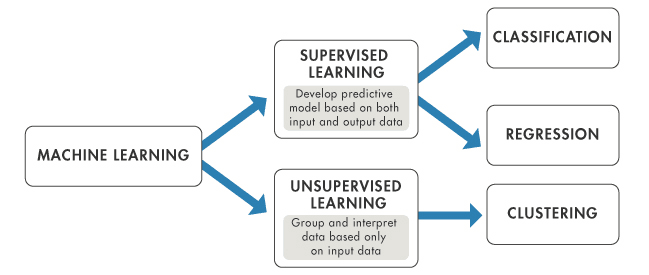### Classification

Classification is a type of supervised machine learning where algorithms learn from the data to predict an outcome or event in the future. For example:

A bank may have a customer dataset containing credit history, loans, investment details, etc. and they may want to know if any customer will default. In the historical data, we will have Features and Target.

• Features will be attributes of a customer such as credit history, loans, investments, etc.
• Target will represent whether a particular customer has defaulted in the past (normally represented by 1 or 0 / True or False / Yes or No.

Classification algorithms are used for predicting discrete outcomes, if the outcome can take two possible values such as True or False, Default or No Default, Yes or No, it is known as Binary Classification. When the outcome contains more than two possible values, it is known as Multiclass Classification. There are many machine learning algorithms that can be used for classification tasks. Some of them are:

• Logistic Regression
• Decision Tree Classifier
• K Nearest Neighbor Classifier
• Random Forest Classifier
• Neural Networks

### Regression

Regression is a type of supervised machine learning where algorithms learn from the data to predict continuous values such as sales, salary, weight, or temperature. For example:

A dataset containing features of the house such as lot size, number of bedrooms, number of baths, neighborhood, etc. and the price of the house, a Regression algorithm can be trained to learn the relationship between the features and the price of the house.

There are many machine learning algorithms that can be used for regression tasks. Some of them are:

• Linear Regression
• Decision Tree Regressor
• K Nearest Neighbor Regressor
• Random Forest Regressor
• Neural Networks

Image Source: https://static.javatpoint.com/tutorial/machine-learning/images/regression-vs-classification-in-machine-learning.png

## Supervised vs. Unsupervised Learning

### Type of Data

The main difference between supervised and unsupervised machine learning is that supervised learning uses labeled data. Labeled Data is a data that contains both the Features (X variables) and the Target (y variable).

When using supervised learning, the algorithm iteratively learns to predict the target variable given the features and modifies for the proper response in order to "learn" from the training dataset. This process is referred to as Training or Fitting. Supervised learning models typically produce more accurate results than unsupervised learning but they do require human interaction at the outset in order to correctly identify the data. If the labels in the dataset are not correctly identified, supervised algorithms will learn the wrong details.

Unsupervised learning models, on the other hand, work in an autonomous manner to identify the innate structure of data that has not been labeled. It is important to keep in mind that validating the output variables still calls for some level of human involvement. For instance, an unsupervised learning model can determine that customers who shop online tend to purchase multiple items from the same category at the same time. However, a human analyst would need to check that it makes sense for a recommendation engine to pair Item X with Item Y.

### Use-cases

There are two prominent use-cases for supervised learning i.e. Classification and Regression. In both the tasks a supervised algorithm learns from the training data to predict something. If the predicted variable is discrete such as “Yes” or “No”, 1 or 0, “Fraud”, or “No Fraud”, then a classification algorithm is required. If the predicted variable is continuous like sales, cost, salary, temperature, etc., then the Regression algorithm is required.

Clustering and Anomaly Detection are two prominent use-cases in unsupervised learning. To learn more about Clustering, check out this article. If you would like to dive deeper into Unsupervised Machine Learning, check out this interesting course by DataCamp. You will learn how to cluster, transform, visualize, and extract insights from unlabeled datasets using scikit-learn and scipy.

### Goals

The objective of supervised learning is to forecast results for new data based on a model that has learned from labeled training dataset. The kind of outcomes you can anticipate are known up front in the shape of labeled data. The objective of an unsupervised learning algorithm is to derive insights from massive amounts of data without explicit labels. Unsupervised algorithms also learn from the training dataset but the training data doesn’t contain any labels.

### Complexity

Supervised machine learning is straightforward relative to unsupervised learning. Unsupervised learning models generally require a large training set in order to yield the desired results, making them computationally complex.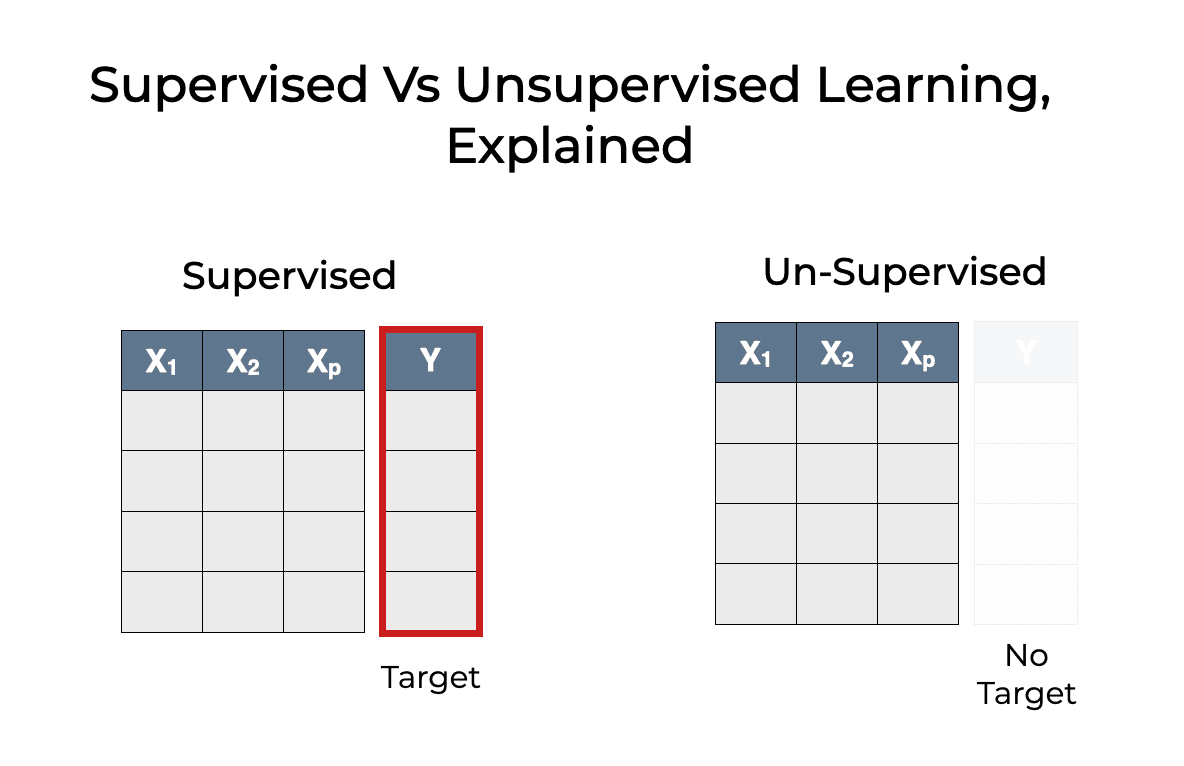Image Image Source: https://www.sharpsightlabs.com/blog/supervised-vs-unsupervised-learning/

## Semi-Supervised Machine Learning

Semi-supervised learning is a relatively new and less popular type of machine learning that, during training, blends a sizable amount of unlabeled data with a small amount of labeled data. Semi-supervised learning is between supervised learning (with labeled training data) and unsupervised learning (unlabeled training data).

Semi-supervised learning offers a lot of real-world applications. There is a dearth of labeled data in many fields. Because they involve human annotators, specialized equipment, or expensive, time-consuming studies, the labels (target variable) could be challenging to get.

Semi-supervised learning has two types: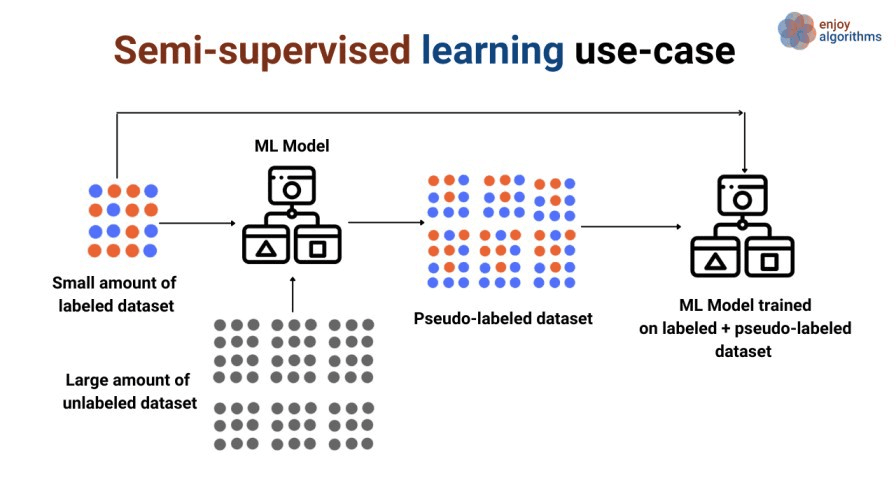Image Source: https://www.enjoyalgorithms.com/blogs/supervised-unsupervised-and-semisupervised-learning

## Supervised Machine Learning Algorithms

In this section we will cover some common algorithms for supervised machine learning:

### Linear Regression

Linear regression is one of the simplest machine learning algorithms available, it is used to learn to predict continuous value (dependent variable) based on the features (independent variable) in the training dataset. The value of the dependent variable which represents the effect, is influenced by changes in the value of the independent variable.

If you recall the “line of best fit” from school days, this is exactly what linear regression is. Predicting a person's weight based on their height is a straightforward example of this concept.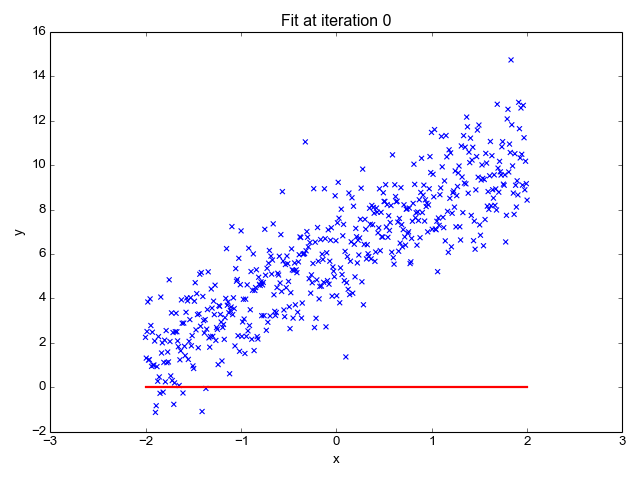Image Source: http://primo.ai/index.php?title=Linear_Regression

 PROS CONS Simple, easy to understand and interpret Easy to overfit Performs exceptionally well for linearly separable data Assumes linearity between features and target variable.

### Logistic Regression

Logistic Regression is a special case of Linear Regression where target variable (y) is discrete / categorical such as 1 or 0, True or False, Yes or No, Default or No Default.  A log of the odds is used as the dependent variable. Using a logit function, logistic regression makes predictions about the probability that a binary event will occur.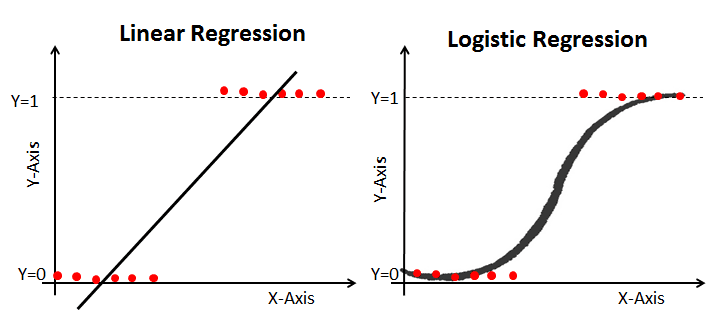PROS CONS Simple, easy to understand and interpret Overfitting Well-calibrated for output probabilities Experience difficulty capturing complex relationships

### Decision Tree

Decision Tree algorithms are a type of probability tree-like structural model that continuously separates data in order to categorize or make predictions depending on the results of the previous set of questions. The model analyzes the data and provides responses to the questions in order to assist you in making more informed choices.

You could, for instance, utilize a decision tree in which the answers Yes or No are used to select a certain species of bird based on data elements like the bird's feathers, its ability to fly or swim, the sort of beak it has, and so on.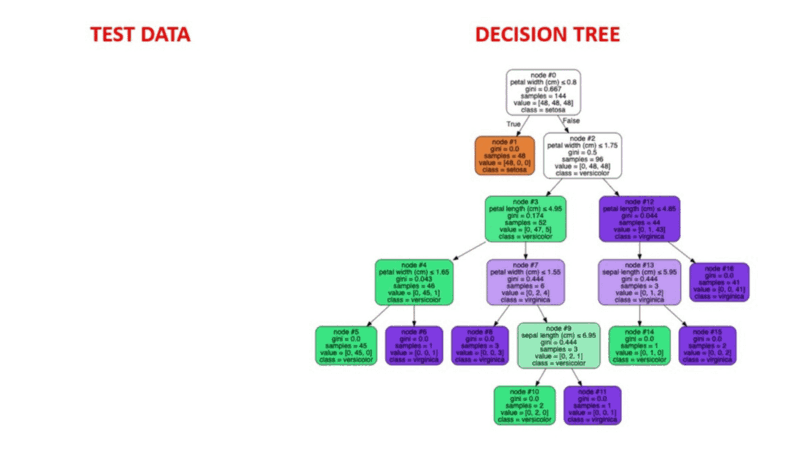PROS CONS Very intuitive and easy to explain Instable - a small change in training data can cause huge differences in prediction. Decision trees don't require a lot of data preparation as some linear models require. Prone to overfitting

To learn more about Machine Learning with Tree-Based Models in Python, check out this interesting course by DataCamp. In this course, you'll learn how to use tree-based models and ensembles for regression and classification using scikit-learn.

### K Nearest Neighbors

K-Nearest Neighbors is a statistical method that evaluates the proximity of one data point to another data point in order to decide whether or not the two data points can be grouped together. The proximity of the data points represents the degree to which they are comparable to one another.

For instance, suppose we had a graph with two distinct groups of data points that were located in close proximity to one another and named Group A and Group B, respectively. Each of these groups of data points would be represented by a point on the graph. When we add a new data point, the group of that instance will depend on which group the new point is closer to.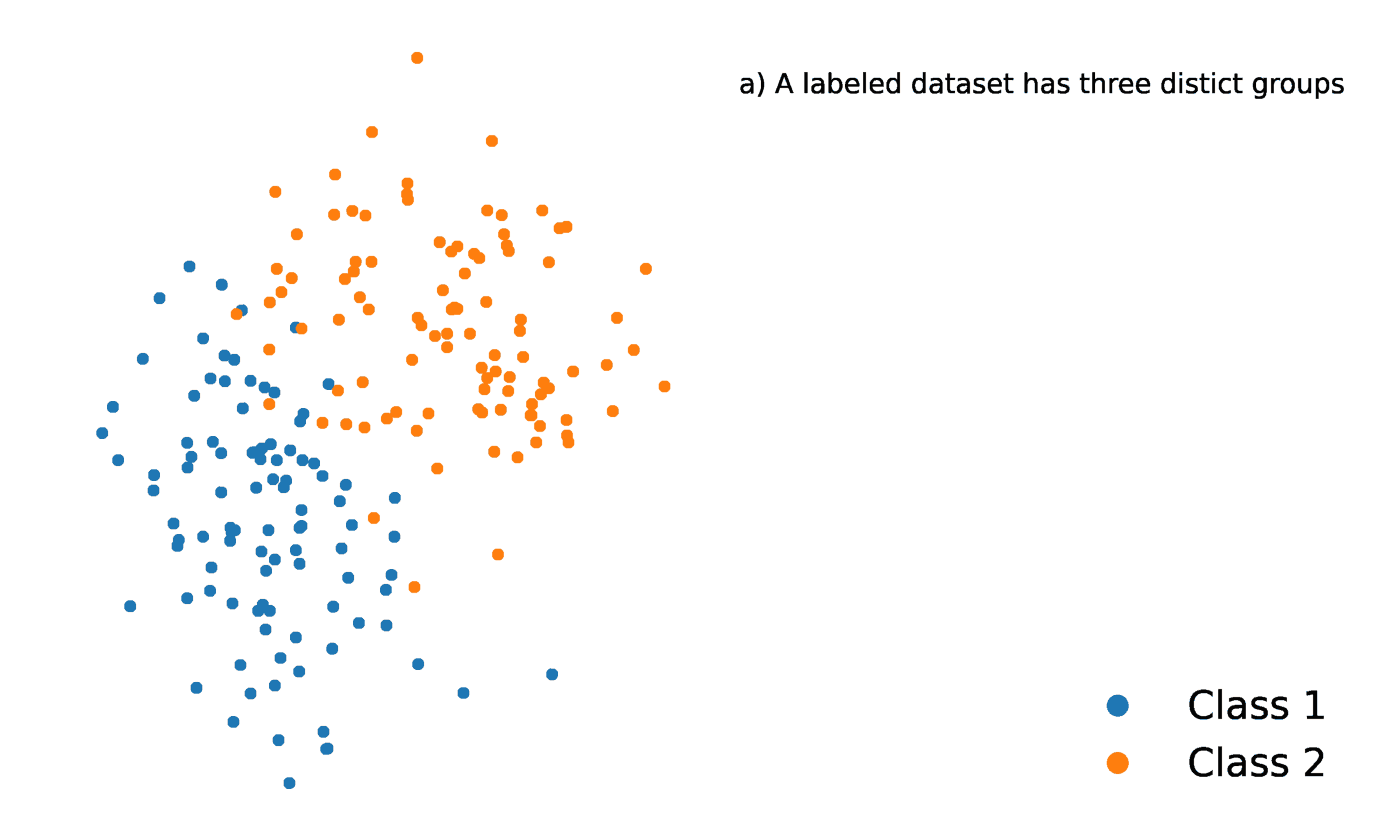PROS CONS Makes no assumption about the data Takes long time for training Intuitive and simple KNN works well with a small number of features but as the numbers of features grow it struggles to predict accurately.

### Random Forest

Random Forest is another example of an algorithm that is built on trees, just like Decision Trees. In contrast to Decision Tree, which only consists of a single tree, Random Forest employs a number of Decision Trees in order to make judgments, creating what is essentially a forest of trees.

It does this by combining a number of different models in order to produce predictions, and it may be used for both classification and regression.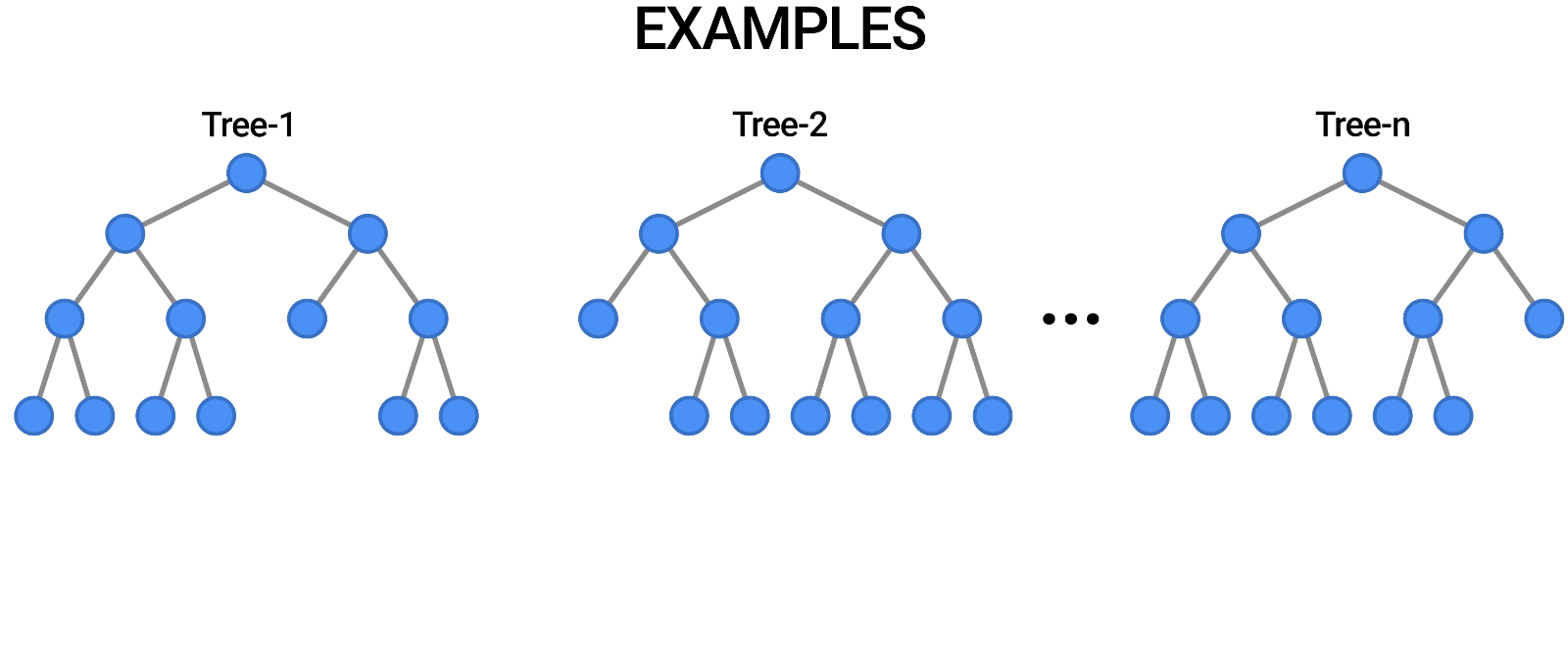PROS CONS Random Forests can handle non-linear relationships in the data easily. Difficult to interpret because of multiple trees. Random Forests implicitly perform feature selection Random Forests are computationally expensive for large datasets.

### Naive Bayes

The Bayes Theorem is a mathematical formula that is used for calculating conditional probabilities, and Naive Bayes is an application of that formula. The probability that an outcome will take place given that another event has already taken place is known as conditional probability.

It makes the prediction that the probabilities for each class belong to a specific class and that the class with the highest probability is the class that is regarded to be the class that is most likely to occur.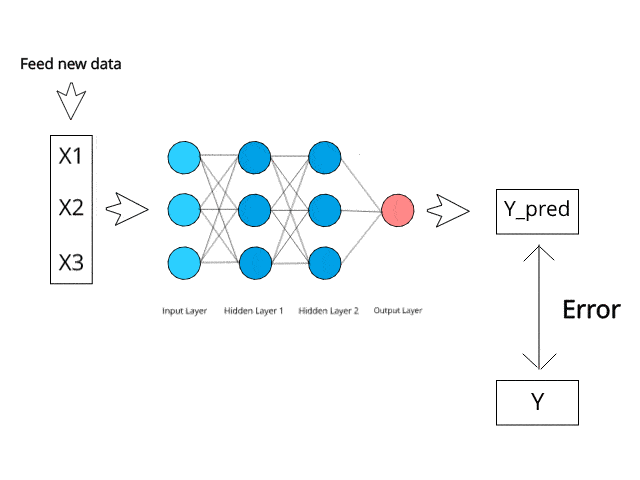PROS CONS The algorithm is very fast. It assumes all features are independent. It is simple and easy to implement The algorithm encounters the "zero-frequency problem," where it gives a categorical variable with zero probability if its category was not present in the training dataset.

## Supervised Machine Learning Python Code Example

In this part we will use scikit-learn in Python to train a Logistic Regression (Classification) model on a fake dataset. Check out the complete Notebook here.

`````````

# create fake binary classification dataset with 1000 rows and 10 features

from sklearn.datasets import make_classification

X, y = make_classification(n_samples = 1000, n_features = 10, n_classes = 2)

# check shape of X and y

X.shape, y.shape

# train test split

from sklearn.model_selection import train_test_split

X_train, X_test, y_train, y_test = train_test_split(X, y, test_size=0.25, random_state=42)

# import and initialize logistic regression model

from sklearn.linear_model import LogisticRegression

lr = LogisticRegression()

# fit logistic regression model

lr.fit(X_train, y_train)

# generate hard predictions on test set

y_pred = lr.predict(X_test)

y_pred

# evaluate accuracy score of the model

from sklearn.metrics import accuracy_score

accuracy_score(y_test, y_pred)

`````````

If you would like to learn supervised machine learning in R, check out this Supervised Learning in R course by DataCamp. In this course you will learn the basics of machine learning for classification in the R programming language.

## Conclusion

Machine learning has completely changed how we conduct business in recent years. A radical innovation that sets machine learning apart from other automation strategies is a departure from rules-based programming. Engineers may use data without explicitly training machines to solve problems in a certain way, thanks to supervised machine learning techniques.

In Supervised machine learning, the expected solution to a problem may not be known for future data, but may be known and captured in a historical dataset and the job of supervised learning algorithms is to learn that relationship from historical data to predict an outcome, event or a value in future.

In this article, we have built a fundamental understanding of what supervised learning is and how it is different from unsupervised learning. We have also reviewed a couple of common algorithms in Supervised learning. However, there are many things we haven’t talked about such as Model Evaluation, Cross-Validation, or Hyperparameter Tuning. If you would like to dig deeper into any of these topics and build your skills further, check out these interesting courses:

### What are two common types of supervised machine learning?.css-1dm0cr5-FAQEntry{-webkit-flex-shrink:0;-ms-flex-negative:0;flex-shrink:0;height:18px;padding-top:6px;-webkit-transform:rotate(0.5turn) translate(21%, -10%);-moz-transform:rotate(0.5turn) translate(21%, -10%);-ms-transform:rotate(0.5turn) translate(21%, -10%);transform:rotate(0.5turn) translate(21%, -10%);-webkit-transition:-webkit-transform 0.3s cubic-bezier(0.85, 0, 0.15, 1);transition:transform 0.3s cubic-bezier(0.85, 0, 0.15, 1);width:18px;}

Classification and Regression are two common types of supervised learning. Classification is used for predicting discrete outcomes such as “Pass” or “Fail”, “True” or “False”, “Default” or “No Default”. Whereas Regression is used for predicting quantity or continuous values such as sales, salary, cost, etc.

### What is the primary difference between supervised and unsupervised learning?.css-qcen5k-FAQEntry{-webkit-flex-shrink:0;-ms-flex-negative:0;flex-shrink:0;height:18px;padding-top:6px;-webkit-transform:none;-moz-transform:none;-ms-transform:none;transform:none;-webkit-transition:-webkit-transform 0.3s cubic-bezier(0.85, 0, 0.15, 1);transition:transform 0.3s cubic-bezier(0.85, 0, 0.15, 1);width:18px;}

The primary difference between supervised and unsupervised learning is the data. For supervised learning you must have labeled data whereas unsupervised learning you do not need labeled data.

### Is time series forecasting supervised learning?

Yes, time series forecasting is supervised learning where we are predicting the next value given the sequence of numbers. It is a supervised learning task.

### Is Natural Language Processing (NLP) supervised or unsupervised learning?

It can be both. It depends on how the problem is framed. For example, predicting the type of text document is most likely to be a supervised task, where on the other hand if the use-case is to group similar documents together based on the text contained in the document, this specific use-case is known as Topic Modeling which is completely unsupervised.

### Is Clustering supervised or unsupervised learning?

For clustering tasks you do not need labeled data hence it is an unsupervised learning.

### Are there different algorithms for supervised and unsupervised learning?

Yes, the algorithms are completely different.

### What are some common algorithms for supervised learning?

Linear Regression, Decision Tree, Neural Networks, Support Vector Machine are some common algorithms for supervised learning.

### .css-1531qan{-webkit-text-decoration:none;text-decoration:none;color:inherit;}Machine Learning with scikit-learn

Beginner
4 hr
317K
Learn how to build and tune predictive models and evaluate how well they'll perform on unseen data.
See Details

### Machine Learning with Tree-Based Models in Python

Beginner
5 hr
68K
In this course, you'll learn how to use tree-based models and ensembles for regression and classification using scikit-learn.

### Understanding Machine Learning

Beginner
2 hr
145.6K
An introduction to machine learning with no coding involved.
See More
Related

### Top Machine Learning Use-Cases and Algorithms

Machine learning is arguably responsible for data science and artificial intelligence’s most prominent and visible use cases. In this article, learn about machine learning, some of its prominent use cases and algorithms, and how you can get started.Vidhi Chugh

15 min### 17 Top MLOps Tools You Need to Know

Discover top MLOps tools for experiment tracking, model metadata management, workflow orchestration, data and pipeline versioning, model deployment and serving, and model monitoring in production.

### Supervised Machine Learning Cheat Sheet

In this cheat sheet, you'll have a guide around the top supervised machine learning algorithms, their advantages and disadvantages, and use-cases.DataCamp Team

5 min

### Unsupervised Machine Learning Cheat Sheet

In this cheat sheet, you'll have a guide around the top unsupervised machine learning algorithms, their advantages and disadvantages and use cases.DataCamp Team

9 min

### A Complete Guide to Data Augmentation

Learn about data augmentation techniques, applications, and tools with a TensorFlow and Keras tutorial.

### Understanding Data Drift and Model Drift: Drift Detection in Python

Navigate the perils of model drift and explore our practical guide to data drift monitoring.
See MoreSee More